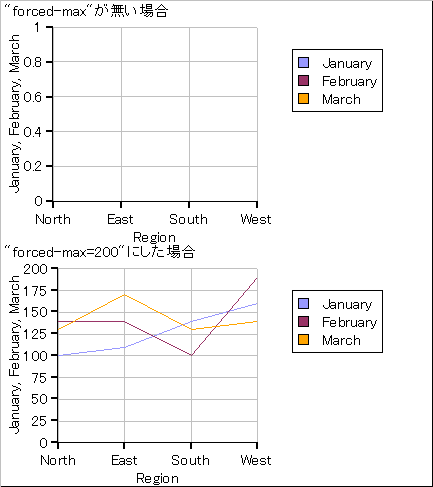# グラフY軸の最大値設定に関して

【ご質問】
グラフY軸のプロパティを以下のように設定したところ、表示される最大値が１になってしまいます。

force-range? = true
forced-min = 0

【回答】
force-range?をtrueに指定した場合は必ずforced-minに加えて
forced-maxも指定する必要があります。

 {curl 6.0,7.0,8.0 applet}{curl-file-attributes character-encoding = “shift-jis”} {import * from CURL.GUI.CHARTS} {let records:RecordSet =    {RecordSet        {RecordFields            {RecordField “Region”, domain = String},            {RecordField “M1”, caption = “January”, domain = int},            {RecordField “M2”, caption = “February”, domain = int},            {RecordField “M3”, caption = “March”, domain = int}        },        {RecordData Region = “North”, M1 = 100, M2 = 140, M3 = 130 },        {RecordData Region = “East”, M1 = 110, M2 = 140, M3 = 170},        {RecordData Region = “South”, M1 = 140, M2 = 100, M3 = 130},        {RecordData Region = “West”, M1 = 160, M2 = 190, M3 = 140}    }}{let chart1:LayeredChart =    {LayeredChart        width = 10cm,        height = 6cm,        left-axis = {new {NumericDataSeriesAxis-of double},                        force-range? = true,                        forced-min = 0,                        {ChartDataSeries records, “M1”},                        {ChartDataSeries records, “M2”},                        {ChartDataSeries records, “M3”}                    },        {LineLayer            {ChartDataSeries records, “M1”},            {ChartDataSeries records, “M2”},            {ChartDataSeries records, “M3”},            x-axis-data = {ChartDataSeries records, “Region”}        }    }}{let chart2:LayeredChart =    {LayeredChart        width = 10cm,        height = 6cm,        left-axis = {new {NumericDataSeriesAxis-of double},                        force-range? = true,                        forced-min = 0,                        forced-max = 200,                        {ChartDataSeries records, “M1”},                        {ChartDataSeries records, “M2”},                        {ChartDataSeries records, “M3”}                    },        {LineLayer            {ChartDataSeries records, “M1”},            {ChartDataSeries records, “M2”},            {ChartDataSeries records, “M3”},            x-axis-data = {ChartDataSeries records, “Region”}        }    }} {VBox    {text “forced-max”が無い場合},    chart1,    {text “forced-max=200”にした場合},    chart2}チャートに使用するデータ（レコードセット）から最大値を取得して

[グラフ]-[LayeredChart 軸]-[軸の範囲の制御]
の項と、APIリファレンスの
[CURL.GUI.CHARTS]-[NumericAxisMixin-of]
の項内のメソッド[set-forced-range]の項をご参照ください。